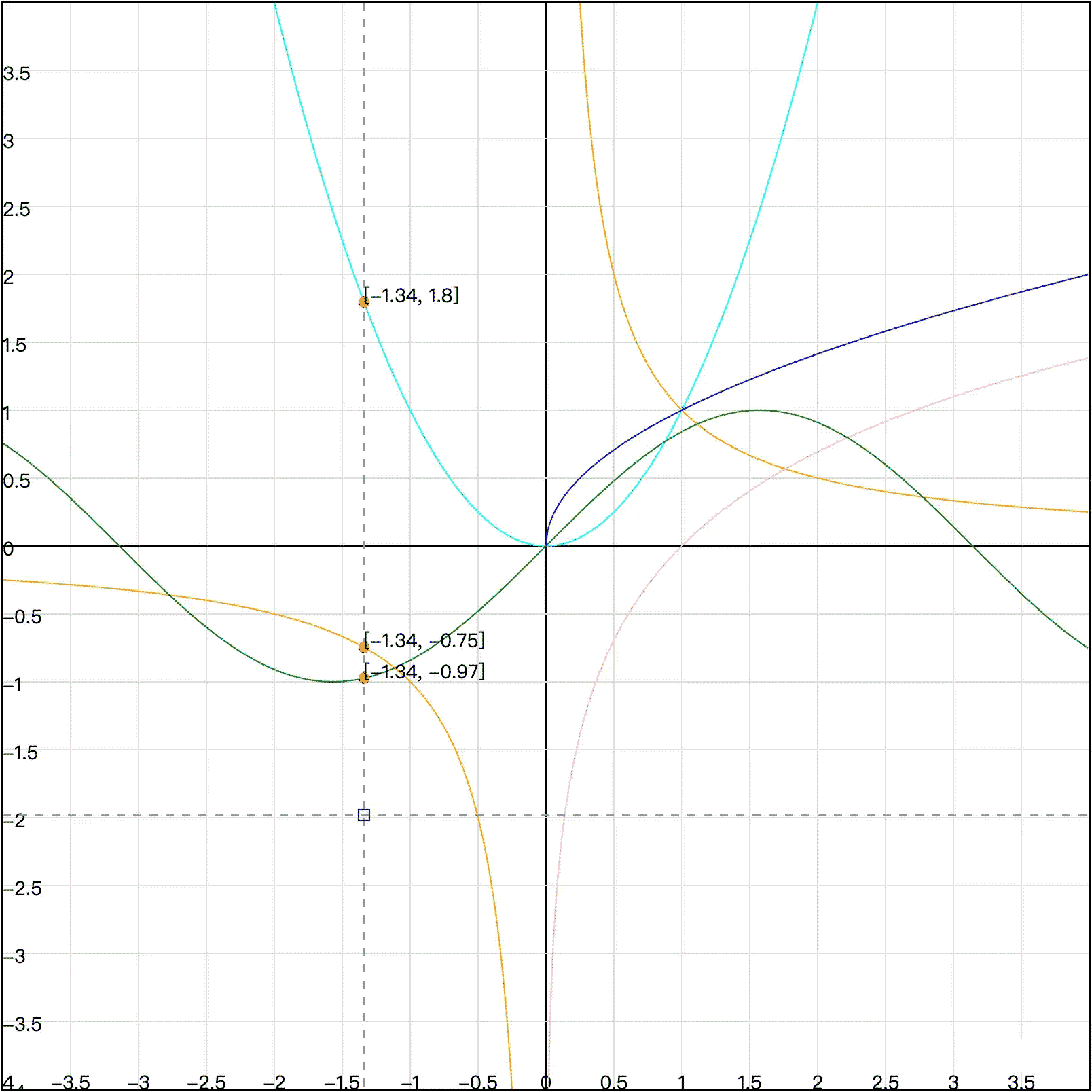﻿ JS前端使用canvas动态绘制函数曲线示例详解_JavaScript_脚本之家
JavaScript# JS前端使用canvas动态绘制函数曲线示例详解

## 前言• 绘制坐标系
• 绘制多条函数曲线
• 绘制辅助线和坐标点
• 支持平移、缩放
• 支持动态绘制曲线
• 使之高清 下面就来一一攻克它们，如果你在电脑前可以顺便瞅瞅示例代码（传送门），不依赖任何库哦。

## 第一步：绘制坐标系

### 2、不是传入多少网格数就是多少网格

```/**
* 计算每一个网格的宽高大小，注意并不是 gridCount 是多少就会有多少网格
* 因为我们的坐标刻度通常都是 10、5、1、0.5、0.1 这个样子，大部分都是以 2、5、10 的倍数
* @param len x 或 y 轴代表的长度
* @param gridCount 网格个数
*/
calcGridSize(len: number, gridCount: number): number[] {
let gridWidth = 1;
// 应该保留几位小数，避免浮点数
let fixedCount = 0;
// 事实上，要是图方便的话，你也可以直接用 unitX 来当做网格大小，不过记得要取整
let unitX = len / gridCount;
// 而这里呢，我们需要找到离 unitX 最近的（稍微偏整数的）值
// 首先要找到离 unitX 最近的 10 的整数倍，如 0.01、0.1、1、10、100
while (gridWidth < unitX) {
gridWidth *= 10
}
while (gridWidth / 10 > unitX) {
gridWidth /= 10
fixedCount++;
}
// 看看能不能再划分一次，如（/5 得到 0.02、0.2、20）、（/2 得到 0.05、5、50）
if (gridWidth / 5 > unitX) {
gridWidth /= 5;
fixedCount++;
} else if (gridWidth / 2 > unitX) {
gridWidth /= 2;
fixedCount++;
}
// 因为 x 轴长度和 y 轴的长度是一样的，所以可以这样赋值
return [gridWidth, gridWidth, fixedCount];
}
```

### 3、如何让坐标原点位于画布中心```drawGrid() {
const { width, height, leftX, rightX, leftY, rightY, xLen, yLen, gridCount, ctx2d } = this;
ctx2d?.save();
// 注意这里我们是将网格数作为配置项，而不是网格的宽高大小
const [gridWidth, gridHeight, fixedCount] = this.calcGridSize(xLen, gridCount);
// 由于计算会产生浮点数偏差，所以要控制下小数点后面的数字个数
this.fixedCount = fixedCount;
// 从左到右绘制竖线
for (let i = Math.floor(leftX / gridWidth); i * gridWidth < rightX; i++) {
// 绘制像素点 / 整个画布宽度 = 实际 x 值 / 实际表示的 x 轴长度
const x = (i * gridWidth - leftX) / xLen * width;
// i = 0 就说明是 y 轴，颜色加深
const color = i ? '#ddd' : '#000';
this.drawLine(x, 0, x, height, color)
this.fillText(String(this.formatNum(i * gridWidth, this.fixedCount)), x, height, this.fontSize, TextAlign.Center);
}
// 绘制横线也是和上面一样的方法，就是要注意画布的 y 轴向下，需要用 height 减一下，或者用 scale(1, -1);
for (let j = Math.floor(leftY / gridHeight); j * gridHeight < rightY; j++) {
let y = (j * gridWidth - leftY) / yLen * height;
y = height - y;
const color = j ? '#ddd' : '#000';
this.drawLine(0, y, width, y, color);
this.fillText(String(this.formatNum(j * gridHeight, this.fixedCount)), 0, y, this.fontSize, TextAlign.Middle);
}
ctx2d?.restore();
}
// 保留 fixedCount 位小数，整数不补零
formatNum(num: number, fixedCount: number): number {
return parseFloat(num.toFixed(fixedCount));
}
```### 4、刻度总是会有浮点数

• 变成整数在计算（先把数字都乘以10的n次方，最后除以10的n次方）
• 变成字符串来计算（类似于小学数学的计算方式，比如乘法，与个位、十位、百位...分别相乘再相加）

## 第二步：画函数曲线

• 点越多画出来的曲线就越平滑，这里我们可以通过参数 steps 来控制，默认值为 1。
• 计算出来的点可以不需要保存下来，因为你一移动、缩放，值就全变了。
• 记得在绘制每条函数开始前 beginPath 一下，否则会影响到下一条曲线的绘制（比如颜色、线宽）。
• 对于画布外的点我们是不需要绘制的，直接用 moveTo 即可（但不是用 continue 跳过）。
```/** 绘制函数曲线，就是用一段段直线连起来 */
drawFn() {
const { width, height, leftX, leftY, xLen, yLen, steps, ctx2d } = this;
if (!ctx2d) return;
ctx2d.save();
this.fnList.forEach(fn => {
ctx2d.strokeStyle = (fn as any).color;
ctx2d.beginPath();
for (let i = 0; i < width; i += steps) {
// 小小的公式推导：像素点 / 画布宽 = x / 实际表示的 x 轴长度
const x = i / width * xLen + leftX;
let y = fn(x);
if (isNaN(y)) continue;
// 换算到具体绘制点
y = height - (y - leftY) / yLen * height;
// 在画布之外是不用绘制的所以用 moveTo 即可
if (i === 0 || y > height || y < 0) {
ctx2d.moveTo(i, y);
} else {
ctx2d.lineTo(i, y);
}
}
ctx2d.stroke();
});
ctx2d.restore();
}
```

```// 初始状态
ctx2d.save();
// 在这里设置各种状态1
ctx2d.save();
// 在这里设置各种状态2
ctx2d.restore();
// 这里是状态1
ctx2d.restore();
// 这里是初始状态
```

## 第三步：绘制辅助线和交点坐标

```drawSubLine(canvasPos: Point) {
const ctx2d: CanvasRenderingContext2D | null = this.ctx2d;
if (!ctx2d) return;
const { width, height } = this;
const { x, y } = canvasPos;
// 先重新绘制网格和函数曲线
this.draw();
// 绘制辅助线
ctx2d.save();
this.drawLine(x, 0, x, height, '#999',true);
this.drawLine(0, y, width, y, '#999', true);
ctx2d.restore();
// 绘制鼠标点
const centerRectLen: number = 8;
this.strokeRect(x - centerRectLen / 2, y - centerRectLen / 2, centerRectLen, centerRectLen);
const actualPos = this.canvasPosToFnVal(canvasPos);
// 绘制曲线和辅助线的交点坐标
this.handleCrosspoint(actualPos.x);
}
handleCrosspoint(x: number) {
const pointList: Point[] = this.checkCrosspoint(x);
pointList.forEach(point => {
const { x, y } = this.fnValToCanvasPos(point);
this.fillCircle(x, y, 4, 'red');
this.fillText(`[\${this.formatNum(point.x, this.fixedCount + 1)}, \${this.formatNum(point.y, this.fixedCount + 1)}]`, x, y, this.fontSize);
});
}
```

## 第四步：平移

```this.canvas.addEventListener('mousedown', this.handleMouseDown.bind(this), false);
// 也可以把 mouseup 和 mousemove 这两个监听写在 mousedown 的回调中
```

```// 返回距离画布左上角的点
viewportToCanvasPosition(e: MouseEvent): Point {
const { clientX, clientY } = e;
const { top, left } = this.canvas.getBoundingClientRect();
const x = clientX - top;
const y = clientY - left;
return new Point(x, y);
}
// 记录鼠标按下的点
handleMouseDown(e: MouseEvent) {
const canvasPos: Point = this.viewportToCanvasPosition(e);
this.state.startPos = canvasPos;
}
// 计算平移距离，更新 leftX 和 rightX 的值，然后重新绘制
handleMouseMove(e: MouseEvent) {
const canvasPos: Point = this.viewportToCanvasPosition(e);
if (!this.state.startPos) return;
document.body.style.cursor = 'move';
this.state.endPos = canvasPos;
const { width, height, xLen, yLen, state: { startPos, endPos } } = this;
// 算出平移距离
const dx = (endPos.x - startPos.x) / width * xLen;
const dy = (endPos.y - startPos.y) / height * yLen;
// 更新边界值
this.leftX -= dx;
this.rightX -= dx;
this.leftY += dy;
this.rightY += dy;
this.xLen = this.rightX - this.leftX;
this.yLen = this.rightY - this.leftY;
this.draw();
this.state.startPos = canvasPos;
}
// 还原拖拽前的状态
handleMouseUp(e: MouseEvent) {
this.state.startPos = null;
this.state.endPos = null;
document.body.style.cursor = 'auto';
}
```

## 第五步：缩放

```this.canvas.addEventListener('mousewheel', this.handleMouseWheel.bind(this), false);
```

```handleMouseWheel(e: Event) {
e.preventDefault();
const event: WheelEvent = e as WheelEvent;
const canvasPos: Point = this.viewportToCanvasPosition(event);
const { deltaY } = event;
const { leftX, rightX, leftY, rightY } = this;
const scale: number = deltaY > 0 ? 1.1 : 0.9;
if (this.isInvalidVal(scale)) return;
const { x, y } = this.canvasPosToFnVal(canvasPos);
// 注意缩放和平移不一样，轴的左右两边一边是加一边是减
this.leftX = x - (x - leftX) * scale;
this.rightX = x + (rightX - x) * scale;
this.leftY = y - (y - leftY) * scale;
this.rightY = y + (rightY - y) * scale;
this.xLen = this.rightX - this.leftX;
this.yLen = this.rightY - this.leftY;
this.draw();
}
/** 缩放过大过小都没啥意义，所以设置下边界值 */
isInvalidVal(ratio: number): boolean {
const { xLen, yLen, MIN_DELTA, MAX_DELTA } = this;
if (ratio > 1 && (xLen > MAX_DELTA || yLen > MAX_DELTA)) return true;
if (ratio < 1 && (xLen < MIN_DELTA || yLen < MIN_DELTA)) return true;
// 上面的判断为什么不直接 （xLen > MAX_DELTA || yLen > MAX_DELTA || xLen < MIN_DELTA || yLen < MIN_DELTA）这样判断呢？
// 因为如果这样判断你会发现缩放到最大和最小的时候，再继续操作都是无效的。
return false;
}
canvasPosToFnVal(canvasPos: Point): Point {
const { width, height, leftX, leftY, xLen, yLen } = this;
const x = leftX + canvasPos.x / width * xLen;
const y = leftY + canvasPos.y / height * yLen;
return new Point(x, y);
}
```## 第六步：动态绘制曲线

• 动画的时候整个画布应该是不可操作的状态。
• 我们的曲线是一条绘制完再绘制另一条的，而不是从左到右同时绘制每条曲线，当然怎么做都可以。
• 有的曲线左边开始部分没有图像，就会出现等待一段时间后才开始绘制的情况，所以我们应该跳过不用绘制的点。